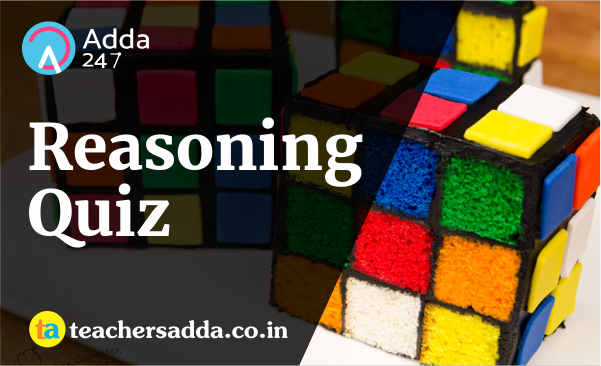The reasoning is the most scoring section in any competitive exam. It also develops or nourishes our critical thinking skills as compared to other subjects. In DSSSB,KVS Exam, As a student to score well in this part, you have to start your preparation now because there is negative marking as well. The more you have prepared, the better you score.

Direction: In the following question, two statements are given each followed by two conclusions I and II. You have to consider the statements to be true even if they seem to be at variance from commonly known facts. You have to decide which of the given conclusions, if any, follows from the given statements.
Q1. Statements:
(I) a R b
(II) b R a

Conclusion:
I. This relation is symmetric.
II. This is a relation.

(a) Conclusion I follows
(b) Conclusion II follows
(c) Neither I nor II follows
(d) Both I and II follows

Directions (2-3): A series is given with one term missing. Choose the correct alternative from the given ones that will complete the series.

Q2. ?, ONM, GFE, XWV
(a) TUV
(b) VUT
(c) GHI
(d) PQR

Q3. RS, ?, BC, GH
(a) WX
(b) XY
(c) UV
(d) GE

Q4. Farhan travels 4 km in the north–east direction and then turns towards the south–east to travel another 3 km. How far is he now from his original position?
(a) 4 km
(b) 6 km
(c) 7 km
(d) 5 km

Q5. In a certain code language, “LOYALTY” is written as “MPZBMUZ”. How is “PROCLAM” written in that code language?
(a) QSPDDBJN
(b) QSPDBMJN
(c) QSPDMBJN
(d) QPSDMBJNQ7. If “S” denotes “multiplied by”, “V” denotes “subtracted from”, “M” denotes “added to” and “L” denotes “divided by”, then 8 V 10 M 96 L 6 S 9 = ?
(a) 140
(b) 142
(c) 134
(d) 144

Q8. Which set of letters when sequentially placed at the gaps in the given letter series shall complete it?
_qr_srq_p_rs_r_p

(a) pqrsss
(b) qprsss
(c) pspqsq
(d) pspqqq

Q9. A man is facing east. He turns 60 degrees in the anticlockwise direction and then again turns another 120 degrees in the same direction. Which direction is he facing now?
(a) East
(b) North-west
(c) South-east
(d) West

Q10. Introducing a woman, Anjali says, “She is the wife of my daughter’s only brother”. How is the woman related to Anjali?
(a) Niece
(b) Daughter-in-law
(c) Sister
(d) Cousin